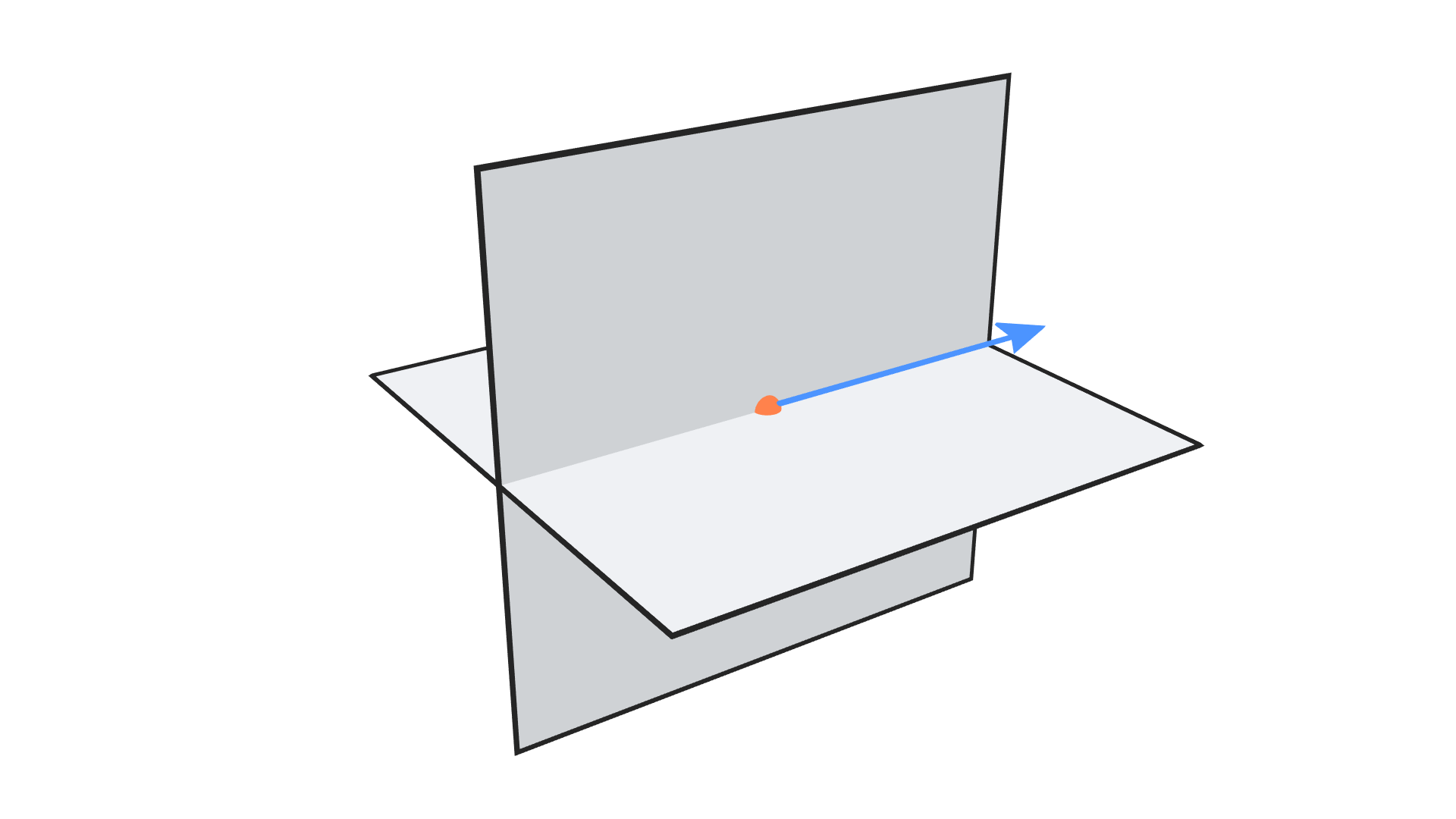# Intersect Plane Plane

## Description

This node returns the intersection line of two input planes. A plane intersect another plane in a straight line. There are multiple representations of this straight line, one of which is the vector form, which represent it using a point and a unit vector defining its direction, this is the form that the node uses. This point could have been in any location along the intersection line, but the node tries to minimize the distance to the origin as much as possible, so it chooses the point along the line that is closest to the origin point.

## IllustrationOrange point is the intersection point and blue vector is the direction vector.

## Inputs

• Plane 1 Point - A point on the first required plane.
• Plane 1 Normal - A unit vector that represent the normal of the first required plane.
• Plane 2 Point - A point on the second required plane.
• Plane 2 Normal - A unit vector that represent the normal of the second required plane.

## Outputs

• Intersection Point - The location of the closest point to the world center that is on the line of intersection of the planes.
• Direction Vector - A unit vector that describe the direction of the line of intersection of the two planes.
• Angle - The angle between input planes in radians.
• Is Valid - A boolean which is True if an intersection was found and False otherwise. If it is False, that means that the planes are parallel or identical.